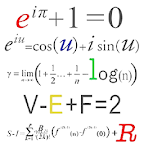Algebra Geometry Formulas, Education

# Algebra Geometry FormulasCategory: Education
Content Rating: Low Maturity
Instalations: 50,000 - 100,000
Version: 1.2
Requirements: Android 2.2 and up
Size: 0.784M
Update: 2013-10-18
Developer: Sun Moon Bytes
Description: Algebra Geometry Formulae is an ideal free app for all students above 12th grade, college graduates, engineering graduates and students preparing for various exams. We have compiled all the algebra, geometry and statistics related formulas to cover all the Math's formulas. The maths topics covered in this free app are: ALGEBRA*Basic Properties and Facts*Factoring and Solving Formulas*Factoring and solving Methods (completing the squares methods etc...)*Functions and Graphs*Common Algebraic ErrorsGEOMETRY*Points and Lines*Angles*Triangles*Quadrilaterals*Polygons*Circles*Coordinate Geometry*Measurement FormulasTRIGONOMETRY*Definitions*Facts and Properties*Formulas and Identities*Unit Circle*Inverse Trigonometric Functions*Law of Sines, Cosines and TangentStatistics*Probability*CombinatoricsTags: learn algebra, geometry, math, free, graphing, geometry textbook, teach geometry, geometry tutor, geometry practice, geometry problems, review geometry, study geometry, geometry prep, geometry cheat sheet, geometry formulas, geometry notes, geometry quicknotes, pre-geometry, algebra 2, algebra tutor, SAT, GRE, CAT, CET, ISEET, NEET, Math, Workout, Practice, Addition, Subtraction, Multiplication, Division, Powers, math games, math game, math workout, maths help,, maths workout, Mathsbrain, maths kids, math tricks, math tutor, math teacher, math test, maths for kids, math drills, math flash cards, math formulas, math facts, math homework, math magic, math maniac, math reference, math ref, math tricks, math skill, math wizard, brain teaser, math problem solving, math logic, SAT, PSAT, GRE, GMAT, ACT, MCAT, maths exam.maths games, maths formulaes, trignometry

Rating: Tags: brain cards cats flash geometry kids logic magic math skill